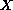Electron. J. Diff. Eqns., Vol. 2003(2003), No. 116, pp. 1-13.

### Existence and stability for some partial functional differential equations with infinite delay Khalil Ezzinbi

Abstract:
We study the existence, regularity, and stability of solutions for some partial functional differential equations with infinite delay. We assume that the linear part is not necessarily densely defined and satisfies the Hille-Yosida condition on a Banach space. The nonlinear term takes its values in space larger than, namely the extrapolated Favard class of the extrapolated semigroup corresponding to the linear part. Our approach is based on the theory of the extrapolation spaces.

Submitted May 29, 2003. Published November 26, 2003.
Math Subject Classifications: 34K20, 34K30, 34K40, 45N05.
Key Words: Hille-Yosida operator, extrapolation spaces, Favard class, regularity, partial functional differential equations, infinite delay, mild solution, linearized stability.

Show me the PDF file (228K), TEX file, and other files for this article.Khalil Ezzinbi Universite Cadi Ayyad Faculte des Sciences Semlalia Departement de Mathematiques B. P. 2390, Marrakech, Morocco email: ezzinbi@ucam.ac.ma